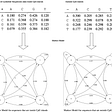# Probability theory: Multivariate random variable, dependent and independent systems of random variables

This is part of the course “Probability Theory and Statistics for Programmers”.

In the article about distribution function, we came to the conclusion that it is possible to find the distribution functions of random variables of a vector by having the distribution function of the system.

Also, we can find probability density of random variable by knowing probability density of the vector.

By using this formulas we can find distribution function or probability density function of a single random variable. But is it possible by knowing distribution functions of separately taken random variables form distribution function of a system? It turns out that in general case this can’t be done. In order to describe a system of random variables we also need to know relationships between variables. We can describe such relationships by using conditional probability distribution.

A conditional probability distribution of random variable X of a system (X,Y) is such distribution, where other random variable Y took specific value y.

In some cases, the dependence between variables can be so close that by knowing the value of one random variable you can find the value of other. In other cases dependence between variables so week that you can call them independent.

Random variable Y is independent of random variable X if the distribution of random variable Y independent from what value take X.

And if Y depends on X:

For independent continuous random variables we can find probability density by multiplying probability densities of separate random variables:

Next part ->

Reach the next level of focus and productivity with increaser.org.

--

--

--

Indie hacker behind increaser.org. More at radzion.com

Love podcasts or audiobooks? Learn on the go with our new app.

## Discrete random variables, PMF, and CDF | Probability theory | Part 3## A brief introduction to the key concepts in Hypothesis Testing## Probability theory: Exponential distribution## A slice of π## A Shot of Scotch #4: Göring Gambit | Chess Openings Explained## What drives stock prices?## Markov Chain Analysis and Simulation using PythonIndie hacker behind increaser.org. More at radzion.com

## A gentle introduction to Random Variables and Probability Distributions## 23.Markov models & Hidden Markov models## What are Descriptive Statistics?